Calculate Depreciation in Excel (Straight Line, Double Declining…)

An asset is defined as a resource with an inherent economic value that is owned by an individual, corporation or country which will yield future economic benefit. Accountants list assets on balance sheets, in order to contribute to increased valuation of the company. Assets can be classified as current assets, fixed assets, financial investment assets or intangible assets. Vehicles, furniture and equipment can be classified as fixed assets. They are listed on a company’s balance sheet and their value depreciated (reduced) as time progresses, in order to give an accurate reflection of their economic value.

Depreciation is a measure of how much the value of an asset decreases over time. A business or individual may buy an asset, for example, a vehicle, a computer or another piece of equipment and then work out the value of that asset over a period of time using depreciation, for accurate reflections on accounting budgets.

Read More: How to Calculate Variance in Excel

Let’s say you bought a laptop four years ago, for \$1 000, that laptop certainly would not be worth that amount in all likelihood today, due to depreciation. Depreciation is divided into two categories namely – the straight line depreciation method which works on the premise that a fixed asset decreases in value proportionally to its useful life, whereas the accelerated depreciation method assumes that the asset loses its value faster in the earlier periods of its lifespan.

Excel has five different depreciation functions. Namely the SLN Function, the SYD Function, the DDB Function, the DB Function and the VDB Function. Each of the depreciation functions utilizes a somewhat different method for calculating the depreciation of an asset.

SLN Excel Function

The simplest of the five is the SLN Function which uses the straight-line depreciation method and thus returns the straight-line depreciation of an asset.

The syntax for the SLN Function is: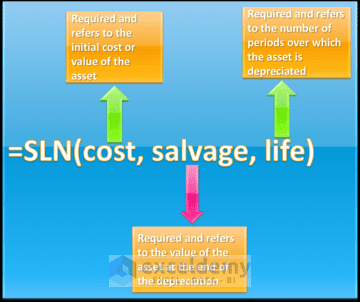The SLN is the easiest of the depreciation functions to use and understand, however, the drawback with this function, is that it can often deliver results that are unrealistic at times. Therefore the other four depreciation functions, which allow for the fact that in reality assets lose more of their value during the initial periods of their lifespan, are utilized.

SYD Excel Function

The SYD Function utilizes the sum-of-years-digits method, in order to calculate the depreciation of an asset over a given time period.

The syntax for the SYD Function is: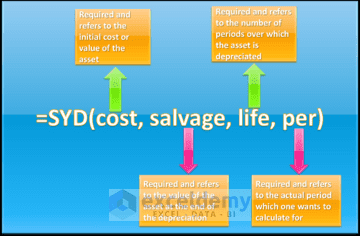The SYD Function is a relatively good choice for depreciation calculations. The one thing to remember though is that the units for the life and per inputs must be the same, in other words, if the useful life is given in years, then per also has to be in years.

Read More: Calculating the Rate an Investment Achieves Using the RRI Function

DDB Excel Function

The DDB Function utilizes the double-declining-balance depreciation method to calculate depreciation as the default, however it can utilize another method if this is specified. It calculates the depreciation of an asset, by doubling the depreciation that the SLN method uses. This results in a very fast reduction of the asset’s value. There is one issue with the DDB Function, however, and that is eventually using this function, an asset can dip below its final value or even be delivered as a negative. The way to handle these situations is that as soon as the DDB given depreciation falls below what the straight-line depreciation would be, one must then discard the DDB results and use the SLN function instead. One can also utilize the VDB depreciation function as an accelerated depreciation alternative. When one utilizes the DDB Function for depreciation calculations, one must be aware that the double-declining balance method results in the depreciation being highest in the first period, and decreasing in successive periods.

The syntax for the DDB Function is: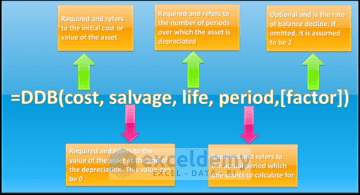DB Excel Function

The DB Function uses the fixed-declining balance method in order to calculate the depreciation of an asset. Utilizing this method means, that an asset’s annual decline value is set at a fixed percentage of its book value.

The syntax for the DB Function is: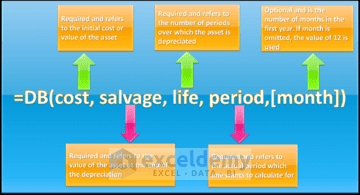Excel VDB Function

The VDB Function is another frequently used accelerated depreciation function. The VDB Function allows one to specify the factor, to multiply the straight line depreciation by and also allows one to calculate depreciation for a specified period or partial periods. This method utilizes a variable declining balance approach as a result of the inputs allowed. It is the most complex of the depreciation functions, but the most useful and allows for partial period calculations.

The syntax of the VDB Function is: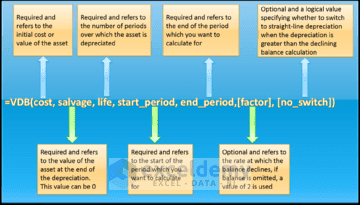So, let’s get started with a few simple examples, to demonstrate when to use the different depreciation functions.

How to Calculate Straight Line Depreciation in Excel with SLN Function

An accountant working on compiling a balance sheet wants to calculate the depreciation for an asset that cost  \$50 000, has a salvage value of \$15 000 and a useful life of 8 years, using the simple SLN Function.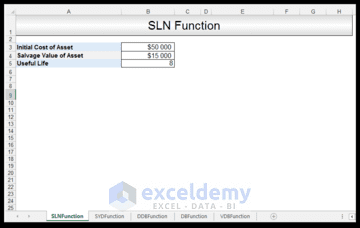1) In order to calculate the SLN, in Cell B6 we input the following formula:

=SLN (B3, B4, B5)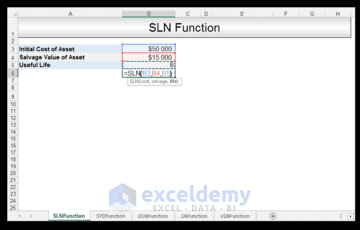2) Upon pressing CTRL-ENTER, a depreciation allowance value of \$4 375 for each year is returned.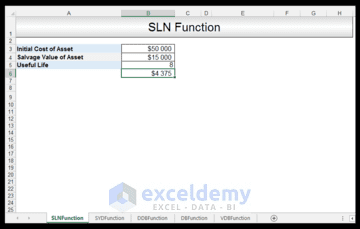Using the SYD Function to Calculate the Depreciation

An accountant working on compiling a balance sheet wants to calculate the depreciation for an asset that cost  \$50 000, has a salvage value of \$15 000 and a useful life of 8 years, for the third year of its lifespan using the SYD Function.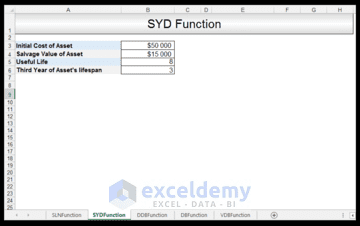1) In order to calculate the SYD, in Cell B7 we input the following formula:

=SYD (B3, B4, B5, B6)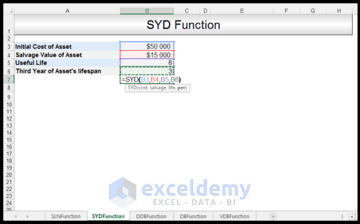2) Upon pressing CTRL – ENTER, a yearly depreciation allowance for year 3 of \$5 833 is delivered.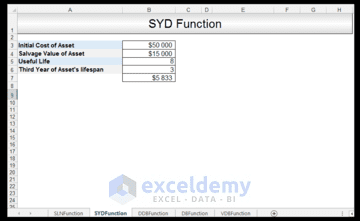As we can see the asset is depreciated much faster using this function, in comparison to the SLN Function. However, one must just be aware that the period value, as in year 3 in this case, should never be greater than the overall lifespan of the asset.

Read More: The PV, FV and PDURATION Excel Functions Tutorial

Using the DDB Function to Calculate the Double Declining Depreciation in Excel

An accountant working on compiling a balance sheet wants to calculate the depreciation for an asset that cost \$50 000, has a salvage value of \$15 000 and a useful life of 8 years, for the third year of its lifespan using the DDB Function. We would like to use the double-declining balance method, so we can omit the factor, since then by default it’s assumed to be 2.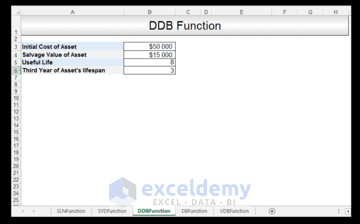1) In order to calculate the DDB, in Cell B7 we input the following formula:

=DDB (B3, B4, B5, B6)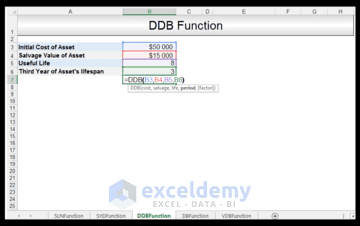2) Upon pressing CTRL-ENTER, a yearly depreciation allowance for year 3, using the double-declining balance method, of \$7 031 is delivered.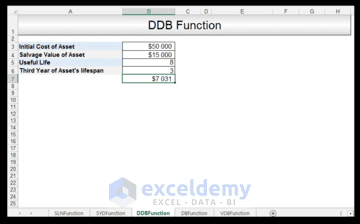Using the DB Function to Calculate the Depreciation

An accountant working on a balance sheet wants to calculate the depreciation for an asset that cost \$50 000, has a salvage value of \$15 000 and a useful life of 8 years, for the third year of its lifespan, with 10 months in its first year using the DB Function.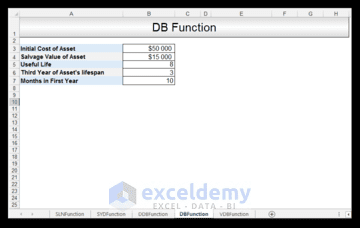1) In order to calculate the DB, in Cell B8 we input the following formula:

=DB (B3, B4, B5, B6, B7)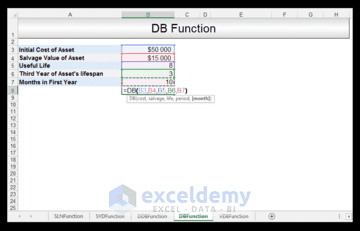2) Upon pressing CTRL-ENTER, a yearly depreciation allowance for year 3 with the first year of 10 months, using the fixed-declining balance method, of \$5 318 is delivered.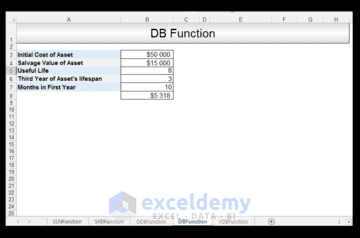Using the VDB Function to Calculate the Depreciation in Excel

Read More: The FVSCHEDULE Excel Function

An accountant working on compiling a balance sheet wants to calculate the depreciation for an asset that cost \$50 000, has a salvage value of \$15 000 and a useful life of 8 years. The depreciation is being calculated for the 48th to 52nd month time frame. A factor of 1.5 is being used. The no_switch value will be set to TRUE, in order to ensure that Excel does not switch to straight-line depreciation even when the depreciation is greater than the declining balance calculation. If the no-switch value is omitted then Excel assumes it is FALSE and switches to the straight-line depreciation method, when the straight-line depreciation is greater than the declining balance depreciation amount. We multiply 8 by 12, in order to get the number of months total for the useful lifespan, which in this case is 96.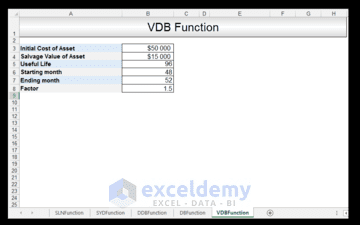1) In order to calculate the VDB, in Cell B9 we input the following formula:

=VDB (B3, B4, B5, B6, B7, B8, TRUE)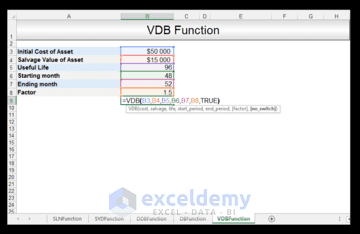2) Upon pressing CTRL-ENTER a value of \$1 433 is returned. This gives the depreciation for the period between the 48th month and the 52nd month at a factor of 1.5.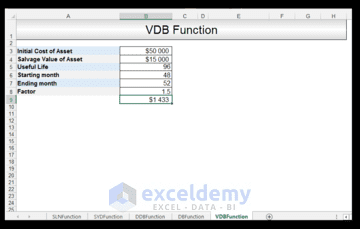Conclusion

Depreciation describes the loss in value of an asset that occurs over time. Accountants are frequently given lists of different kinds of assets for which they have to compute the depreciation for, in order to give a realistic overview of the company’s financial health. There are five different depreciation functions in Excel. The SLN Function is the most simple, however, it can give a slightly unrealistic, overly optimistic view of an asset’s value after a depreciation period. The four other depreciation functions are all accelerated depreciation functions and are preferred at times, for a more comprehensive realistic calculation of an asset’s value after a depreciation period.

Please feel free to comment and tell us which of the depreciation functions you use most often in your own financial spreadsheets.